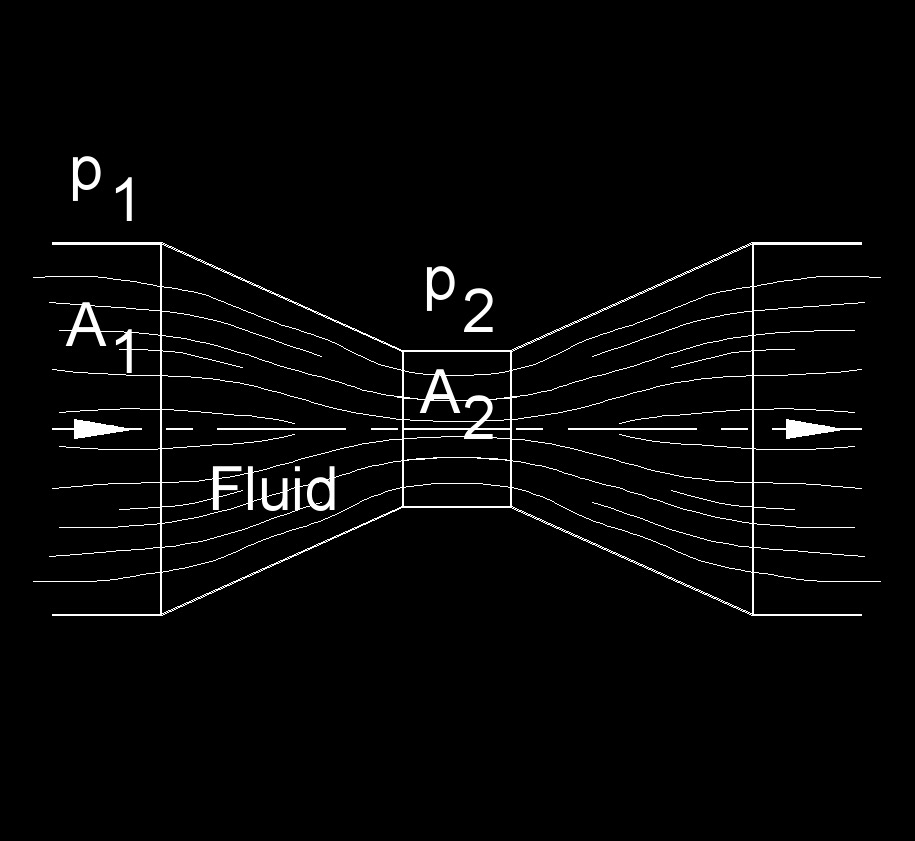# Venturi Meter Flow Rate

Written by Jerry Ratzlaff on . Posted in Fluid DynamicsVenturi meter flow rate measures the velocity of a fluid through a pipe.

## Venturi Meter Flow Rate Formula

 $$\large{ Q = A_2 \sqrt { \frac { {2 \; \left ( p_1 \; - \; p_2 \right)} } { \rho \; \left( 1\; - \; \left( \frac {A_2} {A_1} \right) ^2 \right) } } }$$

### Where:

$$\large{ Q }$$ = flow rate

$$\large{ A_1 }$$ = area 1

$$\large{ A_2 }$$ = area 2

$$\large{ p_1 }$$ = pressure 1

$$\large{ p_2 }$$ = pressure 2

$$\large{ \rho }$$ = density of the fluid

Tags: Equations for Flow